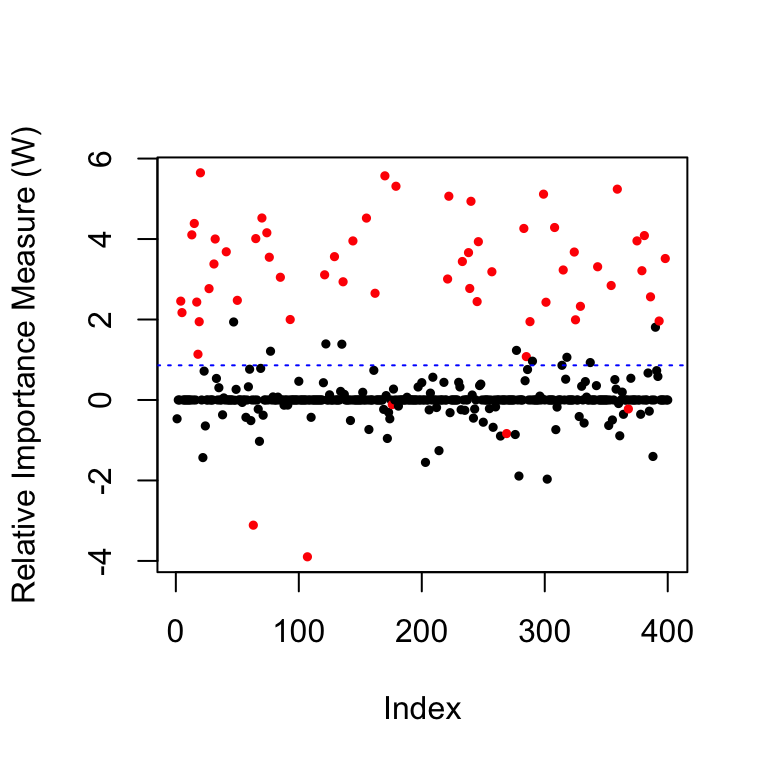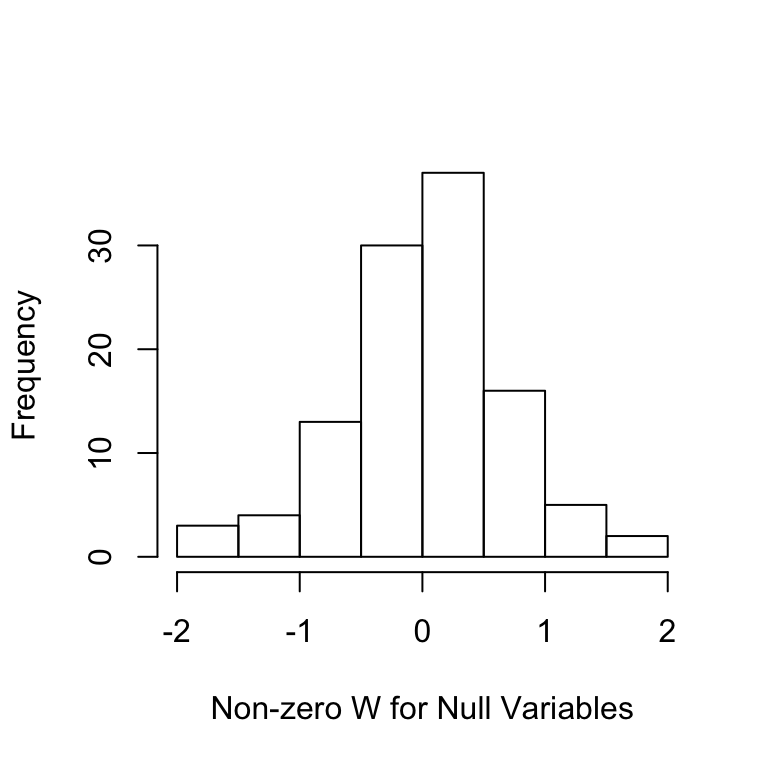This page shows examples of conditional knockoffs for the multivariate gaussian models in Section 3.1 of the accompanying paper, which allows for arbitrary mean and covariance matrices.

library("lattice")
library(knockoff)
source('src/knockoff_measure.R')
source('src/util.R')
source('src/cknock_ldg.R')

In this scenario we assume that the distribution of the covariates belongs to a multivariate Gaussian family with unknown mean and unknown covariance matrix. We will separately look at the cases where the number of observations $$n$$ is greater than 2 times the dimension $$(2p)$$ and where $$n<p$$ but unlabeled data are available.

## Low-Dimensional

We first set up the experiment with $$n>2p$$.

set.seed(2019)
# Problem parameters
p = 400         # number of covariates
n = 3*p         # number of observations
k = 60          # number of non-zero regression coefficients
A = 5           # signal amptitude
nonzero = sample(p, k)
beta = 1 * (1:p %in% nonzero) * sample(c(-1,1),p,rep = T)
# Generate the covariates from a multivariate normal distribution
rho = 0.3
Sigma = rho^abs(outer(1:p,1:p,'-'))      # Covariance matrix
Sigma.chol = chol(Sigma)

# Generate the covariates
X = matrix(rnorm(n * p), n) %*% Sigma.chol

# Generate the response from a linear model
Y = X%*%beta*A/sqrt(n) + rnorm(n)

The conditional knockoffs can be generated by calling the function where the method option refers to how $$\mathbf{s}$$ is computed and mix is used in the simulations of Section 3.1.2 and discussed in Appendix B.1.1.

Xk.cond = cknockoff.ldg(X,method = 'mix')

We can look at the histogram of the original-knockoff correlations for all the $$X_j$$ to make sure they are not too high.

cor.ok=diag(cor(X,Xk.cond))
hist(cor.ok,main='',xlab='Original-Knockoff Correlation')Once we have generated conditional knockoffs, all the usual knockoffs machinery applies.

fdr.level = 0.2
knockoff.stat.fun =  mod.stat.lasso_coefdiff
filter.cond = knockoff.filter(X,Y,
knockoffs = function(x) {
Xk.cond
},
statistic = knockoff.stat.fun,
fdr = fdr.level,offset = 1)
# false positive and false negative
c(fp(filter.cond$selected, beta), fn(filter.cond$selected, beta))
##  0.1538462 0.9166667

As post-diagnostics, we can look at the distribution of the $$W$$ statistics.

coloring=rep(1,p); coloring[nonzero]=2  # the color for the true non-zero locations of beta is red
plot(filter.cond$statistic,ylab='Relative Importance Measure (W)', pch=19,col=coloring,cex=0.5) # plot the statistics used by the knockoff filter abline(h=filter.cond$threshold,col='blue',lty=3)  # indicates the threshold
Ws=filter.cond$statistic[-nonzero];Ws=Ws[Ws!=0] hist(Ws,main='',xlab='Non-zero W for Null Variables')## High-Dimensional with Unlabeled Data In this example, we set up the experiments with a small sample size ($$n<2p$$) but with additional unlabeled data such that the total number of covariate rows is more than $$2p$$. # Problem parameters n = 300 n.u = 2*p # Generate labelled and unlabelled covariate X = matrix(rnorm(n * p), n) %*% Sigma.chol X.u = matrix(rnorm(n.u * p), n.u) %*% Sigma.chol # Generate the response from a linear model Y = X%*%beta*A/sqrt(n) + rnorm(n) We then generate low-dimensional conditional knockoffs for all the covariates but only keep the rows corresponding to the labeled data. Xk.star = cknockoff.ldg(rbind(X,X.u),method = 'mix') Xk.cond = Xk.star[1:n,] filter.cond = knockoff.filter(X,Y, knockoffs = function(x) { Xk.cond }, statistic = knockoff.stat.fun, fdr = fdr.level,offset = 1) # false positive and false negative c(fp(filter.cond$selected, beta),
fn(filter.cond\$selected, beta))
##  0.1707317 0.5666667